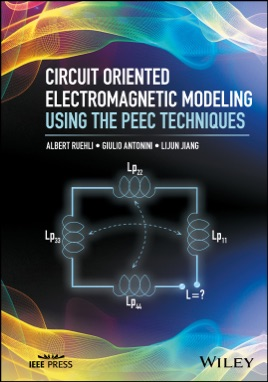• \$124.99

## Publisher Description

Bridges the gap between electromagnetics and circuits by addressing electrometric modeling (EM) using the Partial Element Equivalent Circuit (PEEC) method

This book provides intuitive solutions to electromagnetic problems by using the Partial Element Equivalent Circuit (PEEC) method. This book begins with an introduction to circuit analysis techniques, laws, and frequency and time domain analyses. The authors also treat Maxwell's equations, capacitance computations, and inductance computations through the lens of the PEEC method. Next, readers learn to build PEEC models in various forms: equivalent circuit models, non-orthogonal PEEC models, skin-effect models, PEEC models for dielectrics, incident and radiate field models, and scattering PEEC models. The book concludes by considering issues like stability and passivity, and includes five appendices some with formulas for partial elements.
Leads readers to the solution of a multitude of practical problems in the areas of signal and power integrity and electromagnetic interference Contains fundamentals, applications, and examples of the PEEC method Includes detailed mathematical derivations
Circuit Oriented Electromagnetic Modeling Using the PEEC Techniques is a reference for students, researchers, and developers who work on the physical layer modeling of IC interconnects and Packaging, PCBs, and high speed links.